• Posts tagged "framework"

# Blog Archives

## R语言游戏框架设计

R的极客理想系列文章，涵盖了R的思想，使用，工具，创新等的一系列要点，以我个人的学习和体验去诠释R的强大。

R语言作为统计学一门语言，一直在小众领域闪耀着光芒。直到大数据的爆发，R语言变成了一门炙手可热的数据分析的利器。随着越来越多的工程背景的人的加入，R语言的社区在迅速扩大成长。现在已不仅仅是统计领域，教育，银行，电商，互联网….都在使用R语言。

• 张丹(Conan), 程序员Java,R,PHP,Javascript
• weibo：@Conan_Z
• blog: http://blog.fens.me
• email: bsspirit@gmail.com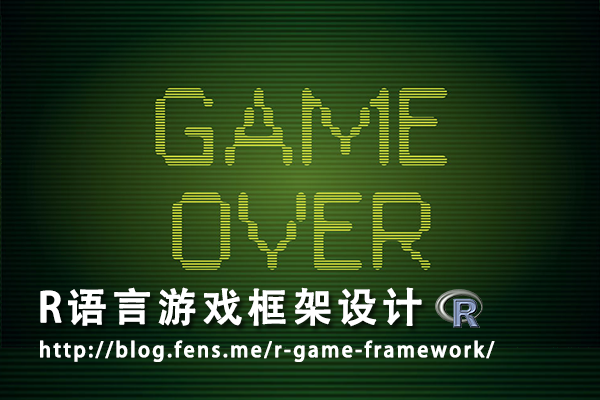1. 贪食蛇的面向对象改造
2. 游戏框架定义
3. 在框架中重新实现贪食蛇游戏

## 1. 贪食蛇的面向对象改造

1.1 定义Snake类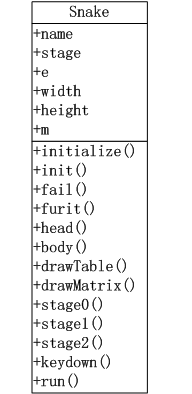• name:游戏的名字
• stage:当前的游戏场景
• e:游戏中的变量，environment类型
• m:游戏地图矩阵
• width:矩阵的宽
• height:矩阵的高

• initialize():构建函数，用于RC类的初始化
• init():给stage1场景初始化游戏变量
• fail():失败查询
• furit():判断并生成水果坐标
• body():生成蛇尾移动坐标。
• drawTable():绘制游戏背景。
• drawMatrix():绘制游戏矩阵。
• stage0():创建开机场景，可视化输出。
• stage1():创建游戏场景，stage1()函数内部，封装了游戏场景运行时的函数，并进行调用。
• stage2():创建结束场景，可视化输出
• keydown():监听键盘事件。
• run():启动函数

1.2 全局函数调用顺序图

1. 通过run()函数启动游戏，进入stage0场景，注册键盘事件。
2. 在stage0场景按任意键切换到stage1场景。
3. init()出始化stage1场景的游戏变量。
4. stage1()运行游戏
5. 当游戏失败fail()或按q键
6. 游戏进行stage2场景，显示游戏结束画面，
7. 按空格键回到stage0重新开始，按q键退出程序。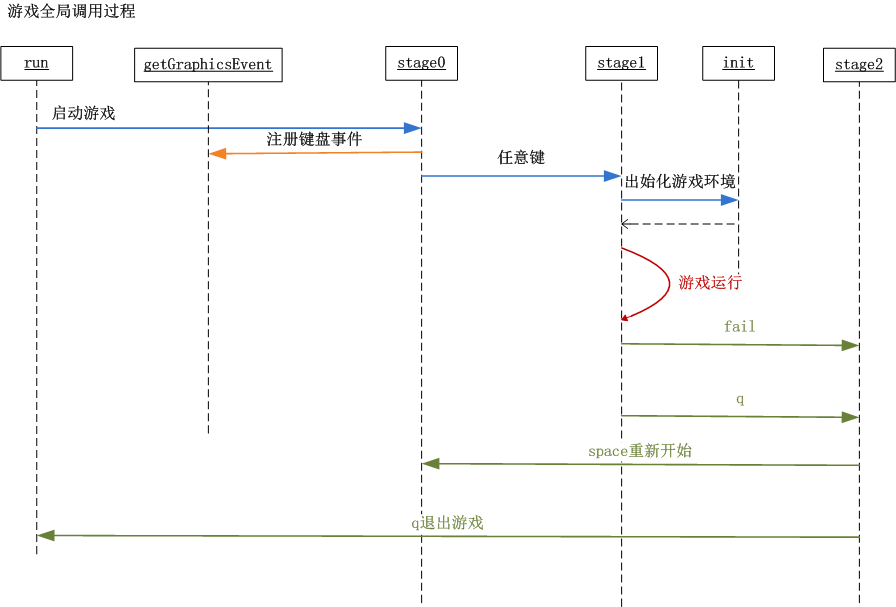1.3 stage1场景游戏环境函数调用顺序图

stage1场景游戏环境函数调用关系。

1. 游戏进入stage1场景，按上下左右(up,down,left,right)方向键操作蛇头的前进路线。
2. furit()函数检查，如果地图上水果被吃掉，生成一个新水果，记录到矩阵中。
4. fail()函数失败检查，no未失败继续，yes失败进行stage2场景。
5. body()函数，蛇身体移动，记录到矩阵中。
6. drawTable()函数，画出游戏背景画布。
7. drawMatrix()函数，画出游戏矩阵。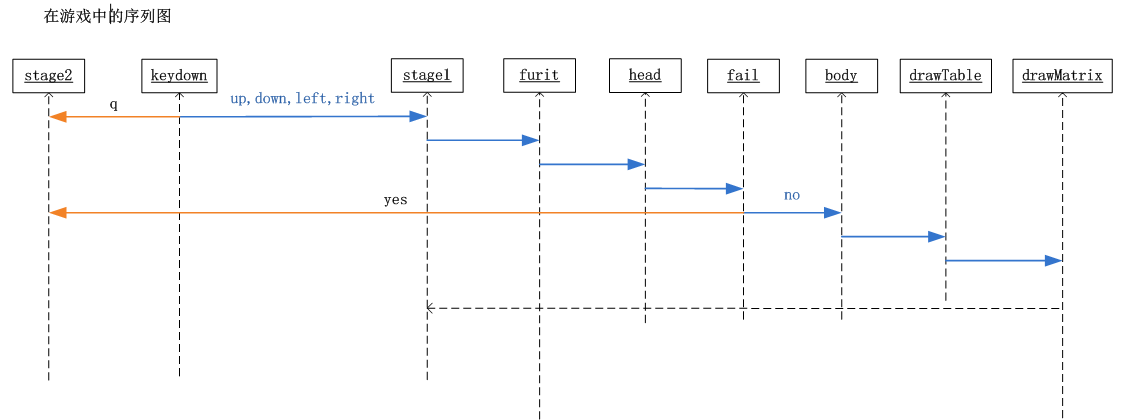## 2. 游戏框架定义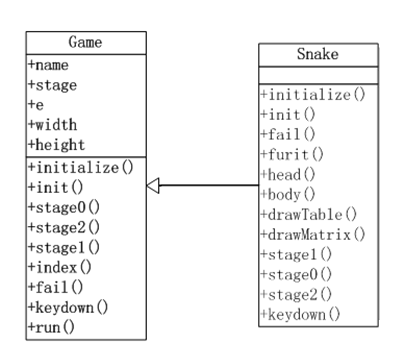• Game类公共属性，包括了所有的Snake类的属性，这是因为这些属性都是全局的，其他的游戏也会用到，而且每个游戏中的属性，可以在e中进行定义。
• Game类公共方法，包括了游戏全局调用的方法，但不包括Snake游戏stage1场景中运行的方法。在Game类的方法中，我们主要实现的都是开发的辅助功能。
• Snake类方法，同样还有Game类的方法，这是用到方法的重写技术。子类的方法，先调用父类的同名方法，然后再执行子类方法里的程序。

## 3. 在框架中重新实现贪食蛇游戏

Game类的代码实现，用R语言的RC面向对象编程进行代码编写。

``````
Game<-setRefClass('Game',

fields=list(
# 系统变量
name="character", # 名字
debug='logical',  # 调试状态
width='numeric',  # 矩阵宽
height='numeric', # 矩阵高

# 应用变量
stage='numeric',  # 场景
e='environment',  # 环境空间变量
m='matrix',       # 数据矩阵
isFail='logical'  # 游戏失败
),

methods=list(

# 构造函数
initialize = function(name,width,height,debug) {
name<<-"R Game Framework"
debug<<-FALSE
width<<-height<<-20   #矩阵宽高
},

# 初始化变量
init = function(){
e<<-new.env()   #环境空间
m<<-matrix(rep(0,width*height),nrow=width)  #数据矩阵
isFail<<-FALSE
},

# 开机画图
stage0=function(){
stage<<-0
init()
},

# 结束画图
stage2=function(){
stage<<-2
},

# 游戏中
stage1=function(default=FALSE){
stage<<-1
if(FALSE){  # 默认游戏中界面
plot(0,0,xlim=c(0,1),ylim=c(0,1),type='n',xaxs="i", yaxs="i")
text(0.5,0.7,label="Playing",cex=5)
}
},

# 矩阵工具
index = function(col) {
return(which(m==col))
},

# 失败操作
fail=function(msg){
print(paste("Game Over",msg))
isFail<<-TRUE
keydown('q')
return(NULL)
},

# 键盘事件，控制场景切换
keydown=function(K){
if(stage==0){ #开机画面
stage1()
return(NULL)
}

if(stage==2){ #结束画面
if(K=="q") q()
else if(K==' ') stage0()
return(NULL)
}
},

# 启动程序
run=function(){
par(mai=rep(0,4),oma=rep(0,4))
stage0()
getGraphicsEvent(prompt="Snake Game",onKeybd=function(K){
if(debug) print(paste("keydown",K))
return(keydown(K))
})
}
)
)
``````

Snake类的代码实现，继承Game类，并实现贪食蛇游戏的私有方法。

``````
# 引用game.r文件
source(file="game.r")

# Snake类，继承Game类
Snake<-setRefClass("Snake",contains="Game",

methods=list(

# 构造函数
initialize = function(name,width,height,debug) {
callSuper(name,width,height,debug) # 调父类

name<<-"Snake Game"
},

# 初始化变量
init = function(){
callSuper()  # 调父类

e\$step<<-1/width #步长
e\$dir<<-e\$lastd<<-'up' # 移动方向
e\$lastx<<-e\$lasty<<-2 # 蛇头上一个点坐标
e\$tail<<-data.frame(x=c(),y=c())#初始蛇尾坐标

e\$col_furit<<-2 #水果颜色
e\$col_tail<<-8 #蛇尾颜色
e\$col_path<<-0 #路颜色
e\$col_barrier<<-1 #障碍颜色
},

# 失败检查
lose=function(){
fail("Out of ledge.")
return(NULL)
}

return(NULL)
}
},

# 随机的水果点
furit=function(){
if(length(index(e\$col_furit))<=0){ #不存在水果
idx<-sample(index(e\$col_path),1)

fx<-ifelse(idx%%width==0,10,idx%%width)
fy<-ceiling(idx/height)
m[fx,fy]<<-e\$col_furit

if(debug){
print(paste("furit idx",idx))
print(paste("furit axis:",fx,fy))
}
}
},

# 方向操作
},

# snake body
body=function(){
if(isFail) return(NULL)

m[e\$lastx,e\$lasty]<<-e\$col_path
if(length(index(e\$col_furit))<=0){ #不存在水果
e\$tail<<-rbind(e\$tail,data.frame(x=e\$lastx,y=e\$lasty))
}

if(nrow(e\$tail)>0) { #如果有尾巴
e\$tail<<-rbind(e\$tail,data.frame(x=e\$lastx,y=e\$lasty))
m[e\$tail[1,]\$x,e\$tail[1,]\$y]<<-e\$col_path
e\$tail<<-e\$tail[-1,]
m[e\$lastx,e\$lasty]<<-e\$col_tail
}

if(debug){
}
},

# 画布背景
drawTable=function(){
if(isFail) return(NULL)

plot(0,0,xlim=c(0,1),ylim=c(0,1),type='n',xaxs="i", yaxs="i")

if(debug){
# 显示背景表格
abline(h=seq(0,1,e\$step),col="gray60") # 水平线
abline(v=seq(0,1,e\$step),col="gray60") # 垂直线
# 显示矩阵
df<-data.frame(x=rep(seq(0,0.95,e\$step),width),y=rep(seq(0,0.95,e\$step),each=height),lab=seq(1,width*height))
text(df\$x+e\$step/2,df\$y+e\$step/2,label=df\$lab)
}
},

# 根据矩阵画数据
drawMatrix=function(){
if(isFail) return(NULL)

idx<-which(m>0)
px<- (ifelse(idx%%width==0,width,idx%%width)-1)/width+e\$step/2
py<- (ceiling(idx/height)-1)/height+e\$step/2
pxy<-data.frame(x=px,y=py,col=m[idx])
points(pxy\$x,pxy\$y,col=pxy\$col,pch=15,cex=4.4)
},

# 游戏场景
stage1=function(){
callSuper()

furit()
lose()
body()
drawTable()
drawMatrix()
},

# 开机画图
stage0=function(){
callSuper()
plot(0,0,xlim=c(0,1),ylim=c(0,1),type='n',xaxs="i", yaxs="i")
text(0.5,0.7,label=name,cex=5)
text(0.5,0.4,label="Any keyboard to start",cex=2,col=4)
text(0.5,0.3,label="Up,Down,Left,Rigth to control direction",cex=2,col=2)
text(0.2,0.05,label="Author:DanZhang",cex=1)
text(0.5,0.05,label="http://blog.fens.me",cex=1)
},

# 结束画图
stage2=function(){
callSuper()
info<-paste("Congratulations! You have eat",nrow(e\$tail),"fruits!")
print(info)

plot(0,0,xlim=c(0,1),ylim=c(0,1),type='n',xaxs="i", yaxs="i")
text(0.5,0.7,label="Game Over",cex=5)
text(0.5,0.4,label="Space to restart, q to quit.",cex=2,col=4)
text(0.5,0.3,label=info,cex=2,col=2)
text(0.2,0.05,label="Author:DanZhang",cex=1)
text(0.5,0.05,label="http://blog.fens.me",cex=1)
},

# 键盘事件，控制场景切换
keydown=function(K){
callSuper(K)

if(stage==1){ #游戏中
if(K == "q") stage2()
else {
if(tolower(K) %in% c("up","down","left","right")){
e\$lastd<<-e\$dir
e\$dir<<-tolower(K)
stage1()
}
}
return(NULL)
}
return(NULL)
}
)
)

snake<-function(){
game<-Snake\$new()
game\$initFields(debug=TRUE)
game\$run()
}

snake()
``````